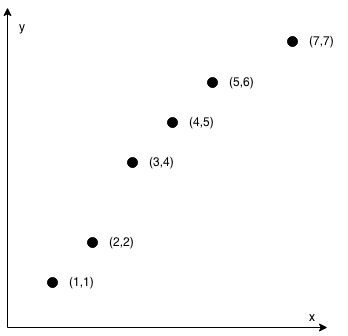You are given an array coordinates, coordinates[i] = [x, y], where [x, y] represents the coordinate of a point. Check if these points make a straight line in the XY plane.

Example 1:Input: coordinates = [[1,2],[2,3],[3,4],[4,5],[5,6],[6,7]] Output: true

Example 2:Input: coordinates = [[1,1],[2,2],[3,4],[4,5],[5,6],[7,7]] Output: false

Constraints:

• 2 <= coordinates.length <= 1000
• coordinates[i].length == 2
• -10^4 <= coordinates[i], coordinates[i] <= 10^4
• coordinates contains no duplicate point.

* Images Source : LeetCode

#### Solution

For any given 3 points (denote the 3rd point as (x, y)), if they are in a straight line, the slopes of the lines from the 3rd point to the 2nd point and the 2nd point to the 1st point must be equal:

(y – y1) / (x – x1) = (y1 – y0) / (x1 – x0)

In order to avoid being divided by 0, use multiplication form:

(x1 – x0) * (y – y1) = (x – x1) * (y1 – y0) => dx * (y – y1) = dy * (x – x1), where dx = x1 – x0 and dy = y1 – y0

#### Output

true

We encourage you to write a comment if you have a better solution or having any doubt on the above topic.